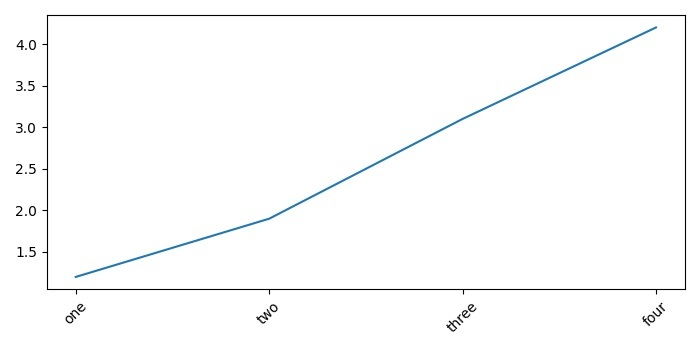# How to set "step" on axis X in my figure in Matplotlib Python 2.6.6?

To set Step on X-axis in a figure in Matplotlib Python, we can take the following Steps −

## Steps

• Create a list of data points, x.
• Add a subplot to the current figure using subplot() method.
• Set xticks and ticklabels with rotation=45.
• To display the figure, use show() method.

## Example

import matplotlib.pyplot as plt
plt.rcParams["figure.figsize"] = [7.00, 3.50]
plt.rcParams["figure.autolayout"] = True
x = [1, 2, 3, 4]
y = [1.2, 1.9, 3.1, 4.2]
plt.plot(x,y)
ax1 = plt.subplot()
ax1.set_xticks(x)
ax1.set_xticklabels(["one", "two", "three", "four"], rotation=45)
plt.show()

## Output